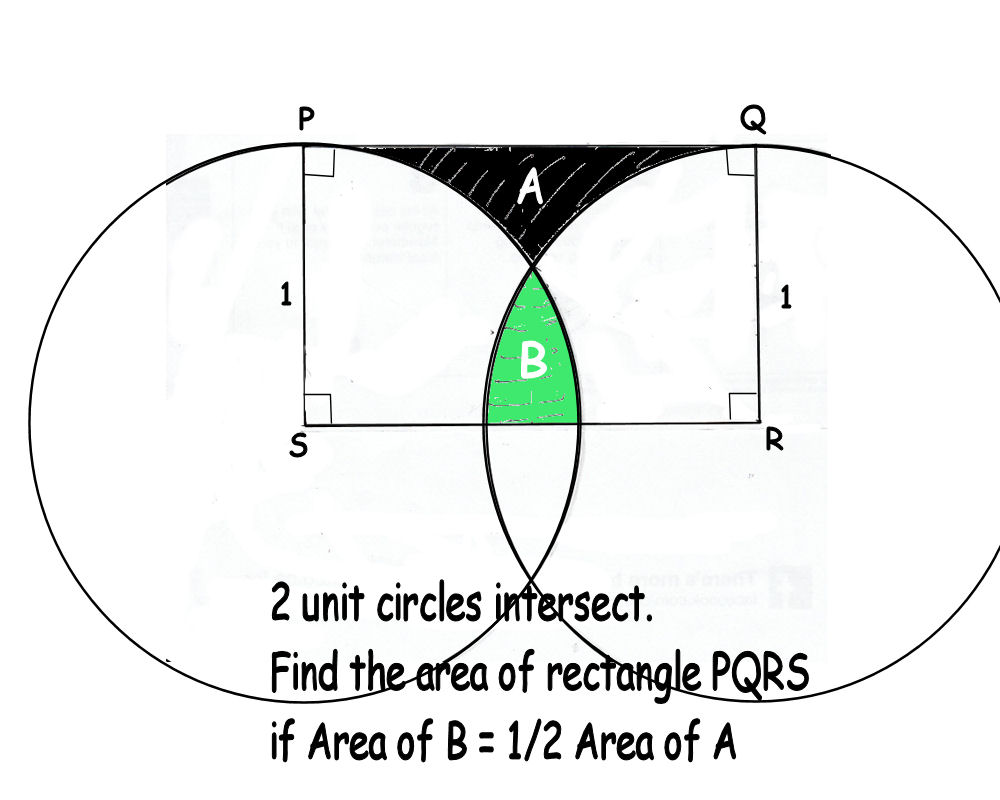# Area of a Rectangle

Geometry Level 5Find the area of the rectangle correct to 3 decimal places.

Hint: The length of SR varies from 2 to 0. At 2, B = 0 and A is maximum, thus B/A = 0. As SR decreases from 2 to 0, B increases and A decreases and B/A increases. You're to find SR and thereby the area of PQRS when B/A = 1/2

×